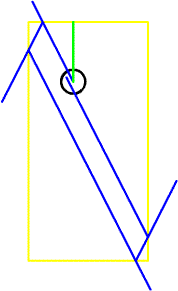.......notes Page 1 Math Home Ideas Home

 16-Apr-1995 Unsolved Problem 16: Does every obtuse triangle admit a periodic orbit for the path of a billiard ball? We assume that the billiard ball bounces off each side so that the angle of incidence equals the angle of reflection. If it hits a vertex, it rebounds along the reflection of its entry path in the angle bisector of the angle at that vertex. The orbit (or trajectory) is periodic, if after a finite number of reflections, it returns to its starting point. Reference: [Croft 1991] Hallard T. Croft, Kenneth J. Falconer, and Richard K. Guy, Unsolved Problems in Geometry. Springer-Verlag. New York: 1991. Page 16. Revision 1 posted 4/25/95. -------------------------------------------------------------------------------- Each week, for your edification, we publish a well-known unsolved mathematics problem. These postings are intended to inform you of some of the difficult, yet interesting, problems that mathematicians are investigating. We do not suggest that you tackle these problems, since mathematicians have been unsuccessfully working on these problems for many years. Problem excerpt from: http://cage.rug.ac.be/~hvernaev/problems/Proble16.htmlWell is an unsolved math problem a challenge to solve or is it not worth trying to solve. You, the reader, be the judge. Even if it is impossible to solve, we, the mathematicians, will have fun trying. And who knows what solutions or techniques will be learned along the way. This billiard ball problem question doesn’t explain it self throughly. My approach is an attempt to find a pattern. Finding a series or way to add the summation of numbers when the ball returns to its start point. This pattern may not occur in the first bounces, but how the ball progresses over time. To do this I have attempted to write a program that would let us see where the ball lands after each bounce and give the coordinates at which this occurs. Here I have finally found a use for the ssine and scosine. Learn more about them here. I take either the ssin or scos depending upon the angle and use it to determine where the ball will hit the table given the angle at which it is hit. Since the size of the table is constant, the length along the axis to one side of the table can be measured. Once the ball strikes the table, the distance to the other side equals the width or length of the table. For example, if the ball is on a four foot by eight foot table the x-axis distance is 4ft. And since that perpendicular distance from the point is known as 4ft we apply the scosine. We take the angle the ball hits the side and determine what length at the given angle it will take to reach 4ft. This length is the scosine. And as long as it doesn’t hit the y-axis rail, we can use it to find the position of the second place the ball hits the rail. This is were the problem begins. I put the computer code in here in its text form. I haven’t put it in a syntax of some computer language yet, but the logic is there. It reads in order from top to bottom. It also has some nested loops that can be confusing. I added comments were I could. I have included the text so that more people can improve upon it. Hopefully I will be able to convert it into C++ or Matlab syntax later.to read notes click here
 References: Learning MatLab 6.5, 1984-2002 The MathWorks, Inc. Analytic Trigonometry with Applications: Sixth Edition, Barnett - Ziegler 1995 PWS Publishing Company
 .......notes Page 1 Math Home Ideas Home